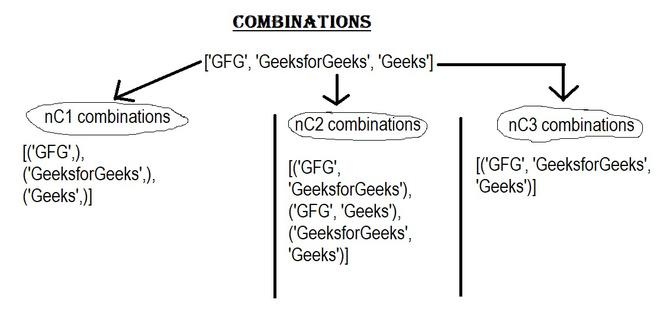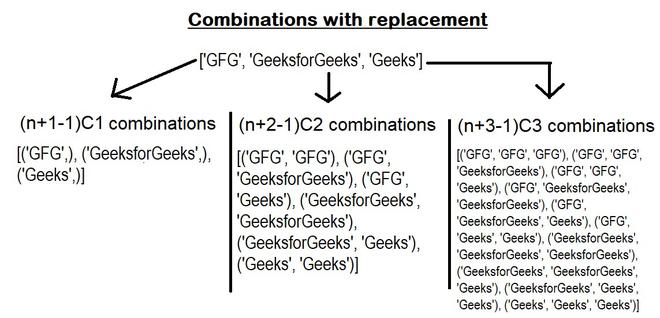Related Articles

# All Combinations For A List Of Objects

• Last Updated : 11 Dec, 2020

Prerequisite: Python Itertools

There are several ways to get all combinations for a list of objects in python. This problem has already a recursive solution. Python has an itertools module that provides two functions named combinations() and combinations_with_replacement() which make our work a lot easier. Below are the two methods:

#### 1. Using itertools.combinations():

Syntax : itertools.combination(iterable, r)

Where r is the length of output tuples.

This function returns subsequences(tuples) of length r from the input iterable. It takes a list of objects and the length of the output tuples(r) as input. But there are a few things to notice about this function like:

• The combination tuples are emitted in lexicographic order. So, if the input iterable is in sorted order the combined output will also be produced in sorted order.
• Elements are treated as unique based on their positions not on their values. So, if the input elements consist of duplicate values there will be duplicate values in the output.
• The number of items returned is nCr = n! / (r! * (n-r)!) when 0 <= r <= n; and zero when r > n.All combinations without replacement

Below is the implementation:

## Python3

 `# code``from` `itertools ``import` `combinations`` ` `# m = list of objects.``# same method can be applied ``# for list of integers.``m ``=` `[``'GFG'``, ``'GeeksforGeeks'``, ``'Geeks'``]``# display``for` `i ``in` `range``(``len``(m)):``  ``print``(``list``(combinations(m, i``+``1``)))`

Output:

```[('GFG',), ('GeeksforGeeks',), ('Geeks',)]
[('GFG', 'GeeksforGeeks'), ('GFG', 'Geeks'), ('GeeksforGeeks', 'Geeks')]
[('GFG', 'GeeksforGeeks', 'Geeks')]```

If the input has duplicate elements:

## Python3

 `# code``from` `itertools ``import` `combinations`` ` `# m = list of objects.``# 1st and 3rd elements are same. ``# same method can be applied ``# for list of integers.``m ``=` `[``'GFG'``, ``'GeeksforGeeks'``, ``'GFG'``]`` ` `# output : list of combinations.``for` `i ``in` `range``(``len``(m)):``  ``print``(``list``(combinations(m, i``+``1``)))`

Output:

```[('GFG',), ('GeeksforGeeks',), ('GFG',)]
[('GFG', 'GeeksforGeeks'), ('GFG', 'GFG'), ('GeeksforGeeks', 'GFG')]
[('GFG', 'GeeksforGeeks', 'GFG')]```

#### 2. Using itertools.combinations_with_replacement():

Syntax: itertools.combination_with_replacement(iterable, r)

Where r is the length of output tuples.

This function works same as itertools.combinations(). But this function returns r length subsequences including individual elements repeated more than once. There are also some points to note:

• The combination tuples are emitted in lexicographic order. So, if the input iterable is in sorted order the combined output will also be produced in sorted order.
• Elements are treated as unique based on their positions not on their values. So, if the input elements consist of duplicate values there will be duplicate values in the output.
• The number of items returned is (n+r-1)! / r! / (n-1)! when n > 0.Combinations with replacement.

Below is the implementation:

## Python3

 `# code``from` `itertools ``import` `combinations_with_replacement`` ` `# m = list of objects.``# same method can be applied ``# for list of integers.``m ``=` `[``'GFG'``, ``'GeeksforGeeks'``, ``'Geeks'``]`` ` `# output : list of combinations.``for` `i ``in` `range``(``len``(m)):``  ``print``(``list``(combinations_with_replacement(m, i``+``1``)))`

Output:

[(‘GFG’,), (‘GeeksforGeeks’,), (‘Geeks’,)]
[(‘GFG’, ‘GFG’), (‘GFG’, ‘GeeksforGeeks’), (‘GFG’, ‘Geeks’), (‘GeeksforGeeks’, ‘GeeksforGeeks’), (‘GeeksforGeeks’, ‘Geeks’), (‘Geeks’, ‘Geeks’)]
[(‘GFG’, ‘GFG’, ‘GFG’), (‘GFG’, ‘GFG’, ‘GeeksforGeeks’), (‘GFG’, ‘GFG’, ‘Geeks’), (‘GFG’, ‘GeeksforGeeks’, ‘GeeksforGeeks’), (‘GFG’, ‘GeeksforGeeks’, ‘Geeks’), (‘GFG’, ‘Geeks’, ‘Geeks’), (‘GeeksforGeeks’, ‘GeeksforGeeks’, ‘GeeksforGeeks’), (‘GeeksforGeeks’, ‘GeeksforGeeks’, ‘Geeks’), (‘GeeksforGeeks’, ‘Geeks’, ‘Geeks’), (‘Geeks’, ‘Geeks’, ‘Geeks’)]

Attention geek! Strengthen your foundations with the Python Programming Foundation Course and learn the basics.

To begin with, your interview preparations Enhance your Data Structures concepts with the Python DS Course. And to begin with your Machine Learning Journey, join the Machine Learning – Basic Level Course

My Personal Notes arrow_drop_up